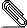# Re: [Snowdrift-discuss] How to manage fees for first real-money operations

```On Sat, Oct 08, 2016 at 12:58:00PM -0400, Patrick 'P. J.' McDermott wrote:
> If you want the amount a project receives to be exactly equal to the
> amount a patron intends to donate, here's the math to determine the
>
> Remember that Stripe's fee is 2.9% + \$0.30, and begin with an equation
> that yields the final donation after fees (\$0.30 less than 100%-2.9%
> of the payment):
>
>     donation = total_payment * (1 - 0.029) - \$0.30
>
> Do some algebra, first adding \$0.30 to both sides:
>
>     donation + \$0.30 = total_payment * (1 - 0.029)
>
> And then divide both sides by 100%-2.9% to isolate total_payment and
> get a formula to calculate it:
>
>                     donation + \$0.30
>     total_payment = ----------------
>                        1 - 0.029```
```
Awesome, thanks! I was caught up calculating the fee, but this way of
looking at it is easier to implement.

I spent some time trying to show that the rounding works out
analytically, but in the end I just brute-forced the calculation on
every possible value. It works (until integer truncation errors occur
up at \$20M).

Plus, I made some cool graphs:
https://www.desmos.com/calculator/xtzs3n4lxq

So, I'll just go ahead with charging enough so that

crowdmatch = net_transaction_balance

I don't really like the lack of an analytical solution, but I'm
satisfied that it actually works.

Brute force code attached.

-Bryan
```
```import Data.Int

stripeFee :: Int32 -> Int32
stripeFee = round . (+ 30) . (* 0.029) . fromIntegral

payment :: Int32 -> Int32
payment = round . (/ (1 - 0.029)) . (+ 30) . fromIntegral

let p = payment c
f = stripeFee p
in p - f /= c

main = do
print (take 1 (filter bad ([1..])))
```
```#include <stdio.h>
#include <limits.h>
#include <math.h>

long int stripe_fee (int c) {
return lrint(c * 0.029 + 30);
}

long int payment (int c) {
return lrint( (c + 30) / (1 - 0.029) );
}

int test (long int c) {
int p, f;
p = payment(c);
f = stripe_fee(p);
return (p - f) != c;
}

int main () {
long int i = 0;
int max = INT_MAX;
for (i = 0; i < max; i++) {
if (test(i)) {
printf("First error at %ld\n", i);
return 0;
}
}
}
```signature.asc
Description: Digital signature

```_______________________________________________
Discuss mailing list
Discuss@lists.snowdrift.coop
https://lists.snowdrift.coop/mailman/listinfo/discuss
```